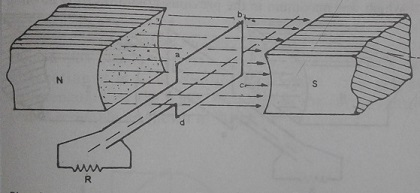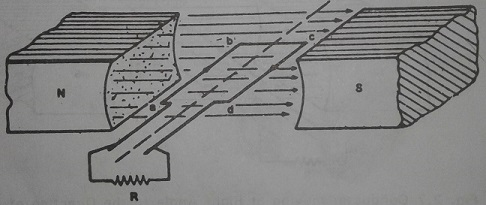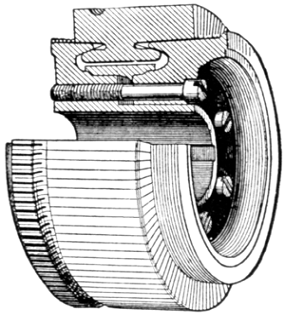# Working of Simple Loop DC Generator

Working Principle of Simple Loop DC Generator

For the purpose of study, a single loop generator is taken. In figure, the single loop generator having a coil with two sides connected to a commutator to which two brushes slide.

The coil is placed in between two permanent magnet in such a way that it can rotate about its axis cutting the flux produced by the permanent magnet.

Five distinct positions of the coil are chosen for the purpose of study. in Fig, the coil side ‘ab' and 'cd' rotate between the north pole and the south pole of a permanent magnet. Assuming the initial position of the coil sides moving parallel to the direction of flux as shown in Fig, no linking of the flux takes place as it does not cut the flux. Hence, no e.m.f. is induced in the conductor.Conductor moving Parallel to the Direction of Flux
Rotating the conductor by 90o clockwise, the conductor travels along the path of a circle and takes a position as shown in Fig. The conductor coil sides 'ab' moves down perpendicular to the flux while the coil side 'cd' moves upwards again perpendicular to the flux. Maximum e.m.f. is induced in the coil and since the sides of the coil arc connected in series, the total e.m.f. is the sum of the e.m.f. of each side of the coil.Conductor moving at Right Angle to the Direction of Flux
If external circuit is complete, the current, in the coil will be in the direction 'b' to 'a' in coil side 'ab’ and 'd' to 'c' in coil side 'cd'. Since the coil sides 'ab' and 'cd' are connected in series, the total e.m.f. is the sum of the e.m.f. induced in each coil side and the direction will be from 'dcba'. Again rotating the coil by another 900 (i.e., 180° from initial position), the coil occupies a position as shown in Fig. The conductor coil sides 'ab' and 'cd' moves parallel to the magnetic flux. Since it does not cut the magnetic flux, no e.m.f. is induced in it. Hence, the e.m.f. which was maximum in the previous position drops down to zero.Conductor moving Parallel to the Direction of Flux
If the position of the coil sides 'ab’ and 'cd' are moved by rotating it further by 90o (i.e., 270° from initial position), it occupies a place as shown in Fig. The coil sides having rotated by 90° and the motion of the coil side 'all' is upwards perpendicular to the magnetic flux while the coil side 'cd' is downwards perpendicular to the magnetic flux. The e.m.f. induced in the coil sides are maximum but since the direction of motion of the conductors are opposite to that shown in Fig, the sign of the maximum e.m.f. induced and the resultant current is also opposite. The direction of current is from 'a' to 'b' in conductor 'ab' and similar from 'c' to 'd' in conductor 'cd'.Figure
Rotating the coil sides by another 90° (i.e., 360° from the initial position) bringing the conductors to the position shown in Fig, the coil travels parallel to the flux and as such no e.m.f. is induced in it. This position also refers to the initial position. The e.m.f. which was negative maximum would drop down to zero. It should be noted that since the conductor moves in the path of a circle and the change in the magnitude of e.m.f. also is smooth following sine law. Since the e.m.f. has a positive quantity and a negative quantity, the e.m.f. is said to alternate from a positive to negative quantity. Therefore, it is called alternating e.m.f. or alternating current. If a graphical representation of the e.m.f. produced in the coil is shown, it would resemble as shown in Fig.

The representation at each stage of rotation of conductor is shown in Fig below.
From the above, it is seen that the e.m.f. produced within the coil is alternating in nature and to convert to direct current a commutator is used. A commutator is a device by which current flows in single direction in the external circuit. The- simplest commutator for the above two pole generator is split ring and is represented in Fig. With the use of commutator the negative portion of the induced e.m.f. is converted into positive such that the current in the external circuit is in single direction. In Fig, two distinct positions of the conductor representing the positions shown in Fig and are shown with an external circuit. Note carefully the flow of current from the coil to the external circuit even though the current in coil side 'ab' and 'cd' change for every 180° rotation of the conductor. The graphical representation of e.m.f. in the external circuit is shown in Fig.

Such unidirectional current is called direct current in short d.c. A generator which gives d.c. in the external circuit is called d.c. generator. Necessarily it uses a commutator.

FUNCTIONS OF SPLIT RING AND COMMUTATOR:

When the coil rotates, the e.m.f. induced in the conductor is of alternating in nature. The current in the conductor coil is the result of the e.m.f. produced in the conductor. Since the coil rotates, there should be a method of drawing the current for the external circuit from a rotating coil to, a stationary terminal. There are three methods of achieving this object. They are :

a. By using a split ring when the generator is of two pole
b. By using a commutator when the generator is of multipole
c. By using a slip ring

The split ring and commutator are used in case the current in the external circuit should, be uni-directional, and slip ring is used when the current in the external circuit has to be alternating. Fig. (a) shows a split ring while Fig (b) shows the commutator. In both the cases, two or four carbon brushes slide over it collecting the current from the rotating conductor. Commutators are used for d.c. machine as well as repulsion motors.(a) Commutator(b) Split Ring
A commutator consists of number of bars, an equal number of mica segments, and an iron core consisting of two end rims and a connecting shell on which the bars and mica segments are placed. Towards the bottom, the bars are partly cut out on both sides in the shape of a V for holding the commutator together by rings. Mica segments are used between bars to prevent adjacent bars from touching. The armature windings are connected to the commutator segments to enable the e.m.f. generated to be collected to a stationary terminal. Fig. shows the commutator assembly.Commutator Assembly